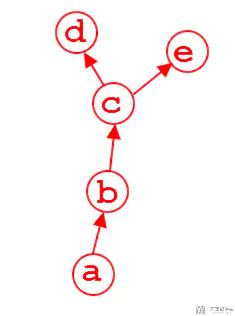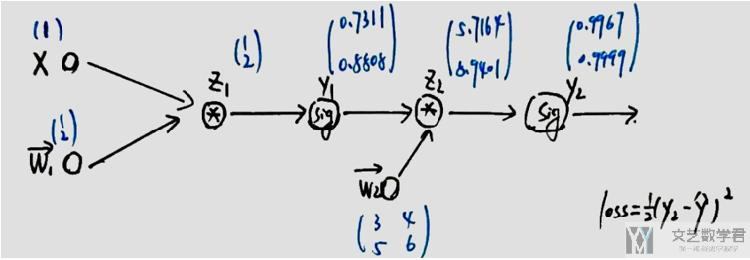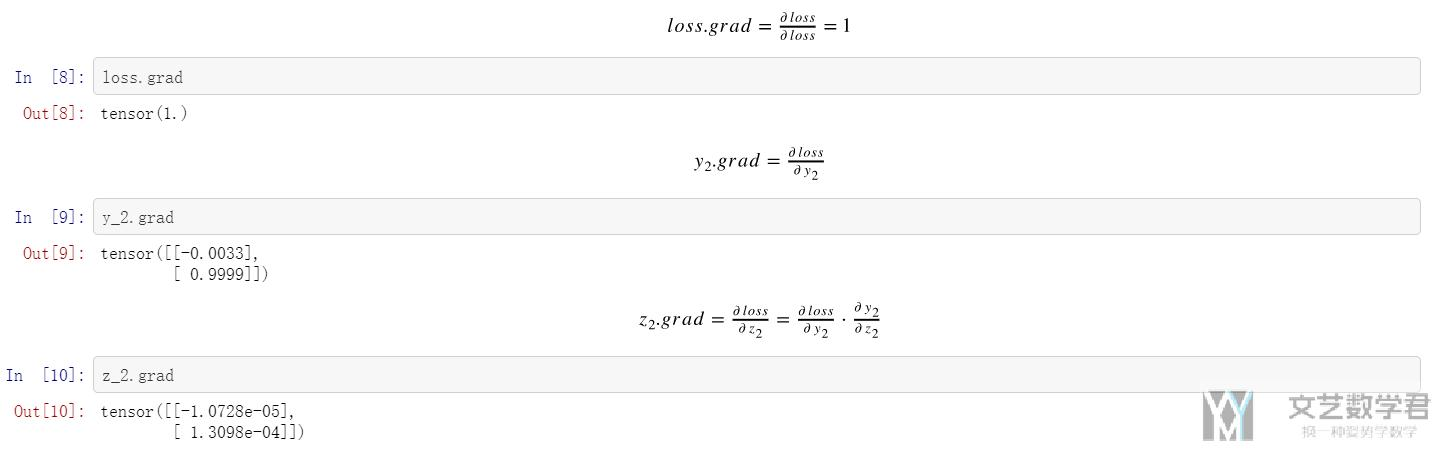# Pytorch入门教程04-非叶子节点梯度保存

• A+

## 简介• hook

### 参考资料1. def forwrad(x, y, w1, w2):
2.     # 其中 x,y 为输入数据，w为该函数所需要的参数
3.     z_1 = torch.mm(w1, x)
5.     y_1 = torch.sigmoid(z_1)
7.     z_2 = torch.mm(w2, y_1)
9.     y_2 = torch.sigmoid(z_2)
11.     loss = 1/2*(((y_2 - y)**2).sum())
13.     return loss, z_1, y_1, z_2, y_2

1. # 测试代码
2. x = torch.tensor([[1.0]])
3. y = torch.tensor([[1.0], [0.0]])
4. w1 = torch.tensor([[1.0], [2.0]], requires_grad=True)
5. w2 = torch.tensor([[3.0, 4.0], [5.0, 6.0]], requires_grad=True)
6. # w2 = torch.tensor([[3.0, 1.0], [1.0, 6.0]], requires_grad=True)
7. # 正向
8. loss, z_1, y_1, z_2, y_2 = forwrad(x, y, w1, w2)
9. # 反向
10. loss.backward()  # 反向传播，计算梯度## hook的使用

1. # 我们可以定义一个hook来保存中间的变量
6.     return hook

1. def forwrad(x, y, w1, w2):
2.     # 其中 x,y 为输入数据，w为该函数所需要的参数
3.     z_1 = torch.mm(w1, x)
4.     y_1 = torch.sigmoid(z_1)
5.     z_2 = torch.mm(w2, y_1)
6.     y_2 = torch.sigmoid(z_2)
7.     loss = 1/2*(((y_2 - y)**2).sum())
8.     return loss, z_1, y_1, z_2, y_2

1. # 测试代码
2. x = torch.tensor([[1.0]])
3. y = torch.tensor([[1.0], [0.0]])
4. w1 = torch.tensor([[1.0], [2.0]], requires_grad=True)
5. w2 = torch.tensor([[3.0, 4.0], [5.0, 6.0]], requires_grad=True)
6. # 正向传播
7. loss, z_1, y_1, z_2, y_2 = forwrad(x, y, w1, w2)
8. # hook中间节点
14. # 反向传播
15. loss.backward()

6. """
7. tensor([[1.2243e-04],
8.         [7.8005e-05]])
9. tensor([[0.0006],
10.         [0.0007]])
11. tensor([[-1.0728e-05],
12.         [ 1.3098e-04]])
13. tensor([[-0.0033],
14.         [ 0.9999]])
15. tensor(1.)
16. """

• 微信公众号
• 关注微信公众号
•• QQ群
• 我们的QQ群号
•